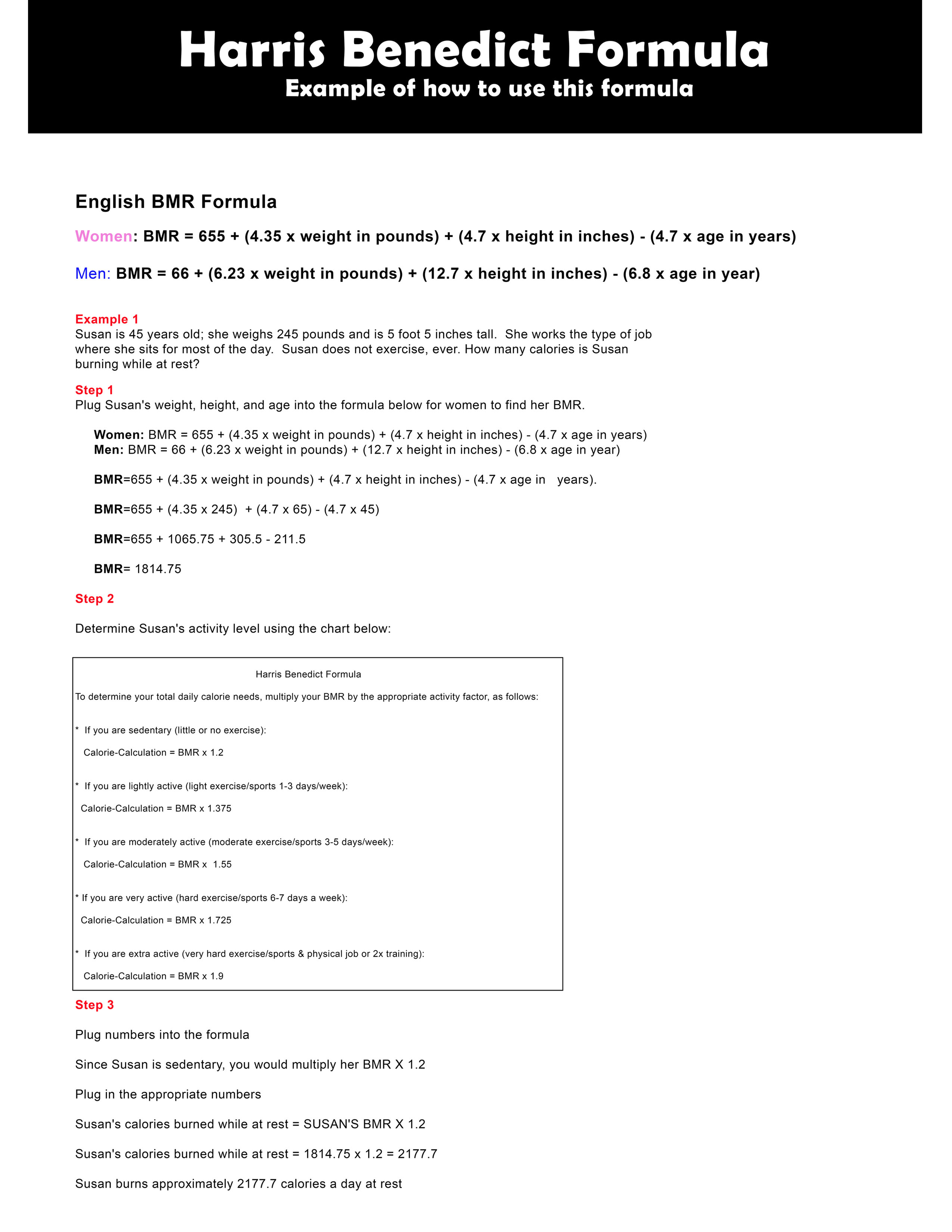# Harris Benedict Formula Example

Here's a Harris Benedict Formula example of how to determine the amount of calories (BMR rate) you burn while resting.

WARNING: Use this equation only as an estimate.

Example 1

Susan is 45 years old; she weighs 245 pounds and is 5 foot 5 inches tall. She works a job where she sits most of the day. She never exercises. Based on these statistics, how many calories is Susan daily?

Step 1

Plug Susan's weight (245lbs), height (5 foot 5 inches), and age (45 yrs) into the formula below for women to find her BMR.

 English BMR Formula Women: BMR = 655 + (4.35 x weight in pounds) + (4.7 x height in inches) - (4.7 x age in years)Men: BMR = 66 + (6.23 x weight in pounds) + (12.7 x height in inches) - (6.8 x age in year)

BMR=655 + (4.35 x weight in pounds) + (4.7 x height in inches) - (4.7 x age in   years).

BMR=655 + (4.35 x 245)  + (4.7 x 65) - (4.7 x 45)

BMR=655 + 1065.75 + 305.5 - 211.5

BMR= 1814.75

Step 2

Determine Susan's activity level using the chart below

 Harris Benedict Formula To determine your total daily calorie needs, multiply your BMR by the appropriate activity factor, as follows:   *  If you are sedentary (little or no exercise): Calorie-Calculation = BMR x 1.2   *  If you are lightly active (light exercise/sports 1-3 days/week): Calorie-Calculation = BMR x 1.375   *  If you are moderately active (moderate exercise/sports 3-5 days/week): Calorie-Calculation = BMR x  1.55   * If you are very active (hard exercise/sports 6-7 days a week): Calorie-Calculation = BMR x 1.725   *  If you are extra active (very hard exercise/sports & physical job or 2x training): Calorie-Calculation = BMR x 1.9

Step 3

Plug numbers into the formula

Since Susan doesn't exercise and inactive she is sedentary according the chart above. Based on that information, multiply her BMR X 1.2

Plug in the appropriate numbers

Susan's calories burned while at rest = SUSAN'S BMR X 1.2

Susan's calories burned while at rest = 1814.75 x 1.2 = 2177.7

Susan burns approximately 2177.7 calories a day at rest